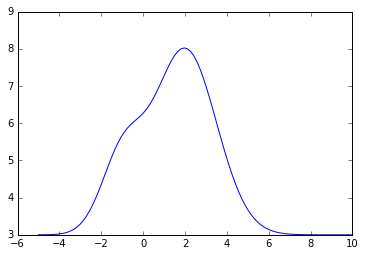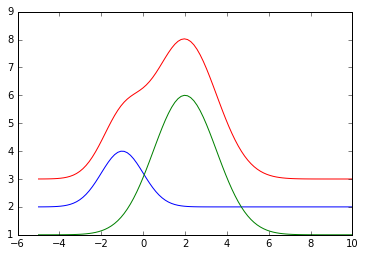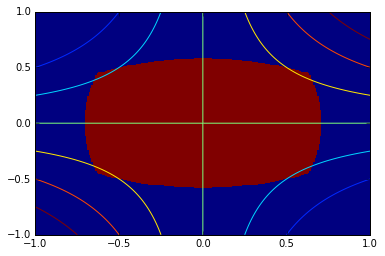# Optimization¶

In :
%matplotlib inline


Suppose you want to optimize (minimize or maximize) a real-valued function (the objective function). The available optimization methods are divided into linear, quadratic and general nonlinear methods based on the objective function. For general nonlinear objective functions one division might be made between those that are functions of a single variable and multivariate objective functions.

The further divisions of the available methods is based on the presence or absence of constraints, on whether the constraints are linear constraints or nonlinear constraints, and whether they are equality or inequality constraints. If the objective function is linear and the constraints are linear equality or inequality constraints one is in the realm of linear programming, one of the oldest and most widely used of the computational optimization areas. The other extreme of general nonlinear objective function with general nonlinear equality and inequality constraints is sometimes called nonlinear programming in contrast and is perhaps the hardest but also very useful in applications.

Finally, one further division is between local and global ooptimization. The methods for linear programming find global optimizer but most of the standard methods of nonlinear optimization are local in nature. Global optimizers are their own big area and techniques like simulated annealing, evolutionary algorithms are sometimes used.

An excellent place to learn about the SciPy functionality available for optimization is the tutorial on the official SciPy document pages and then the accompanying reference guide. The same places also have a good coverage for solving nonlinear equations because some methods for optimization are based on root finding.

## Unconstrained Optimization¶

SciPy optimize package provides a number of functions for optimization and nonlinear equations solving. One such function is minimize which provides a unified access to the many optimization packages available through scipy.optimize. Another way is to call the individual functions, each of which may have different arguments.

## Bird's eye view of unconstrained optimization¶

• Recall Newton's method for solving $f(x) = 0,\; f:\mathbb{R}\rightarrow \mathbb{R}$. Needs derivate $f'(x)$
• Recall Newton's method for solving $f(x) = 0,\; f:\mathbb{R}^n\rightarrow\mathbb{R}^n$. Needs Jacobian $J_f(x)$
• Newton's method for optimization solves $\nabla f(x) = 0,\; f:\mathbb{R}^n\rightarrow\mathbb{R}$. Needs Hessian $H_f(x)$.
• Secant update type methods like BFGS maintain an approximate Hessian.
• Nonlinear conjugate gradient (CG) do not maintain approximate Hessian, hence suitable for large problems.
• Truncated (also known as inexact) Newton methods use few iterations of an iterative solver to solve for the Newton step.

## Single variable¶

See the scipy.optimize tutorial.

## Exercise 1¶

1. Pick a special function or your choice from scipy.special and find minimizers and maximizers in a few intervals using the minimize_scalar in scipy.optimize. Use the argument method='brent'.
2. Modify the above exercise by placing bounds by using minimize_scalar and using the argument method=bounded.

## Nonlinear Least Squares¶

If the unconstrained optimization is a nonlinear least squares optimization without constraints then you can use scipy.optimize.leastsq which is a wrapper for modified Levenberg-Marquardt type methods implemented in a standard well-used old FORTRAN library called MINPACK.

For nonlinear least squares with bounds on the variables use least_squares in scipy.optimize.

Suppose a signal defined on $[-5, 10]$ is measured at 100 points and we known that the signal (i.e. the function) is produced as a sum of two Gaussians with unknown parameters. In particular the signal is known to be of the form

$$A_1 \exp\bigg(-\frac{(x-\mu_1)^2}{2\sigma_1^2}\bigg) + c_1 + A_2 \exp\bigg(-\frac{(x-\mu_2)^2}{2\sigma_2^2}\bigg) + c_2$$

for some unknown paramter values $A_i$, $\mu_i$, $\sigma_i$, $c_i$.

Here is a sample such function with a particular choice of parameters.

In :
from math import sqrt, pi
from numpy import exp, zeros_like, linspace
import matplotlib.pylab as plt

def N(x, A, c, mu, sigma):
return A * exp(-(x-mu)**2/(2.*sigma**2)) + c

def s(x, parameters):
out = zeros_like(x)
for (A, c, mu, sigma) in parameters:
out += N(x, A, c, mu, sigma)
return out
true_parameters = [(2, 2, -1, 1), (5, 1, 2, 1.5)]
x = linspace(-5, 10, 100)
sum = s(x, true_parameters)
plt.plot(x, sum)
plt.figure()
plt.show()<matplotlib.figure.Figure at 0x1172f7fd0>

Since we have produced this signal we know that it is the sum of the following two component functions which are drawn below along with the final signal which is the sume of these 2 Gaussians drawn again for reference.

In :
for (A, c, mu, sigma) in true_parameters:
plt.plot(x, N(x, A, c, mu, sigma))
plt.plot(x, sum)
plt.show()But suppose we did not know these parameter values. How can we determine the parameter values for the two component Gaussians from measuring just the final signal?

It can be done using the nonlinear least squares function scipy.optimize.leastsq. The parameters thus discovered are used to draw the signal as the sum of the discovered Gaussians below.

In :
from scipy.optimize import leastsq

def residuals(parametersflat, y, x):
parameters = [tuple(r) for r in parametersflat.reshape((2,4))]
return y - s(x, parameters)

y_meas = s(x, true_parameters)
# True parameters = [(2, 2, -1, 1), (5, 1, 2, 1.5)]
p0 = [1.9, 2.2, -0.8, 1.3, 4.8, 1.3, 1.8, 1.8]
p = leastsq(residuals, p0, args=(y_meas, x))
fit = s(x, [tuple(r) for r in p.reshape((2,4))])
plt.plot(x, fit)
plt.show()The actual and computed parameters are displayed below.

In :
print 'Actual paramaters'
print true_parameters; print
print 'Computed parameters'
print p

Actual paramaters
[(2, 2, -1, 1), (5, 1, 2, 1.5)]

Computed parameters
[  2.00000000e+00   1.18203679e+04  -1.00000000e+00   1.00000000e+00
5.00000000e+00  -1.18173679e+04   2.00000000e+00   1.50000000e+00]


## Exercise 2¶

1. First copy the example in the tutorial page on nonlinear least squares. Once you have it working, play with increasing the noise in the signal to see how bad can you make it while still recovering reasonable values for the parameters. (You can decide what is reasonable. Plot the reconstructed function and decide whether you find it acceptable.)
2. Try the above exercise using a function constructed as a sume of a number of instances of a parametrized function of your choosing.

See tutorial.

## Exercise 3¶

1. Using the minimum function, compute the minimum of the scalar function $f(x,y) = (x−1)^2 + (y−2)^2 − 3x + 2y + 1$ in two ways: with and without providing the Jacobian.
2. Try to maximize the function $f(x,y) = xy$ subject to the equality constraints \begin{align} 2 x^2 + y^2 &= 1\\ x^2 + 3y^2 &=1 \end{align}

To get a sense of what we're looking at, it may be useful to make a crude 2D plot indicating the constraint region and contours of the objective function. The constraints are the boundary of the constraint region shown.

In :
X, Y = np.meshgrid(np.linspace(-1, 1, 256), np.linspace(-1, 1, 256))

plt.figure()
plt.pcolormesh(X, Y, (2*X**2 + Y**2 <= 1) & (X**2 + 3*Y**2 <= 1))
plt.contour(X, Y, X*Y)
plt.show()## Linear programming¶

Before 2014, SciPy optimize library did not have any linear programming. Now there is scipy.optimize.linprog which we will use. Outside of SciPy you can also consider cvxopt package by S. Boyd and L. Vandenberghe, the authors of the book Convex Optimization. This package can do much more than just linear programming (LP) and has its own sparse matrix formats.

In the optimization community, various languages have been developed for specifying different types of optimization problems at a high level. One widely used language is AMPL (A Mathematical Programming Language) developed at Bell Labs by Robert Fourer, David Gay and Brian Kernighan. Kernighan also co-designed awk in UNIX and is the Kernighan in the famous Kernighan and Ritchie book on C programming. The authors of AMPL have won numerous awards for it from the professional optimization societies.

Let us first solve a simple LP: $$\begin{array}{ll} \mbox{minimize} & 2x_1 + x_2 \\ \mbox{subject to} & -x_1 + x_2 \leq 1 \\ & x_1 + x_2 \geq 2 \\ & x_2 \geq 0 \\ & x_1 -2x_2 \leq 4 \end{array}$$

For reference here is the cvxopt implementation. Your job in the exercise below will be to solve this using scipy.optimize.linprog and compare the solution to the one obtained by cvxopt. Note that a linear program may not have a unique solution.

In :
from cvxopt import matrix
from cvxopt.solvers import lp

A = matrix([[-1.0, -1.0, 0.0, 1.0], [1.0, -1.0, -1.0, -2.0]]) # constraints matrix
b = matrix([1.0, -2.0, 0.0, 4.0 ]) # constraint RHS vector
c = matrix([2.0, 1.0 ]) # cost function coefficients
sol = lp(c, A, b)

     pcost       dcost       gap    pres   dres   k/t
0:  2.6471e+00 -7.0588e-01  2e+01  8e-01  2e+00  1e+00
1:  3.0726e+00  2.8437e+00  1e+00  1e-01  2e-01  3e-01
2:  2.4891e+00  2.4808e+00  1e-01  1e-02  2e-02  5e-02
3:  2.4999e+00  2.4998e+00  1e-03  1e-04  2e-04  5e-04
4:  2.5000e+00  2.5000e+00  1e-05  1e-06  2e-06  5e-06
5:  2.5000e+00  2.5000e+00  1e-07  1e-08  2e-08  5e-08
Optimal solution found.

In :
print(sol['x'])

[ 5.00e-01]
[ 1.50e+00]



Note that the matrix here is not a numpy matrix:

In :
type(A)

Out:
cvxopt.base.matrix

cvxopt matrix can however be easily created from numpy matrices:

In :
import numpy as np

A1 = matrix(np.array([[-1.0, -1.0, 0.0, 1.0], [1.0, -1.0, -1.0, -2.0]]))
type(A1)

Out:
cvxopt.base.matrix

However, the conventions about rows and columns are different. For example, the matrices A and A1 above are transposes of each other even though they were created using similar looking input arguments.

In :
print A
print A1

[-1.00e+00  1.00e+00]
[-1.00e+00 -1.00e+00]
[ 0.00e+00 -1.00e+00]
[ 1.00e+00 -2.00e+00]

[-1.00e+00 -1.00e+00  0.00e+00  1.00e+00]
[ 1.00e+00 -1.00e+00 -1.00e+00 -2.00e+00]



To create A1 to be same as A we should do instead:

In :
A1 = matrix(np.array([[-1.0, -1.0, 0.0, 1.0], [1.0, -1.0, -1.0, -2.0]]).T)
print A
print A1

[-1.00e+00  1.00e+00]
[-1.00e+00 -1.00e+00]
[ 0.00e+00 -1.00e+00]
[ 1.00e+00 -2.00e+00]

[-1.00e+00  1.00e+00]
[-1.00e+00 -1.00e+00]
[ 0.00e+00 -1.00e+00]
[ 1.00e+00 -2.00e+00]



cvxopt also has a sparse matrix format similar to the scipy.sparse.coo_matrix. So it is easy to go back and forth between those:

In :
from cvxopt import spmatrix
from scipy.sparse import coo_matrix

I = [0, 2, 3]; J = [1, 4, 5]; data=[11, 13, 17]
S = spmatrix(data, I, J, tc='d') # tc='d' means typecode is double (float)
S1 = coo_matrix((data, (I, J)), dtype=float)

In :
print S; print S1.todense()

[    0      1.10e+01     0         0         0         0    ]
[    0         0         0         0         0         0    ]
[    0         0         0         0      1.30e+01     0    ]
[    0         0         0         0         0      1.70e+01]

[[  0.  11.   0.   0.   0.   0.]
[  0.   0.   0.   0.   0.   0.]
[  0.   0.   0.   0.  13.   0.]
[  0.   0.   0.   0.   0.  17.]]


## Exercise 4¶

1. Solve the above example using scipy.optimize.linprog.
2. Using scipy.optimize.linprog solve the linear program: maximize $x+y$ subject to \begin{align} 50x + 24y &\le 2400\\ 30x + 33y &\le 2100 \end{align}